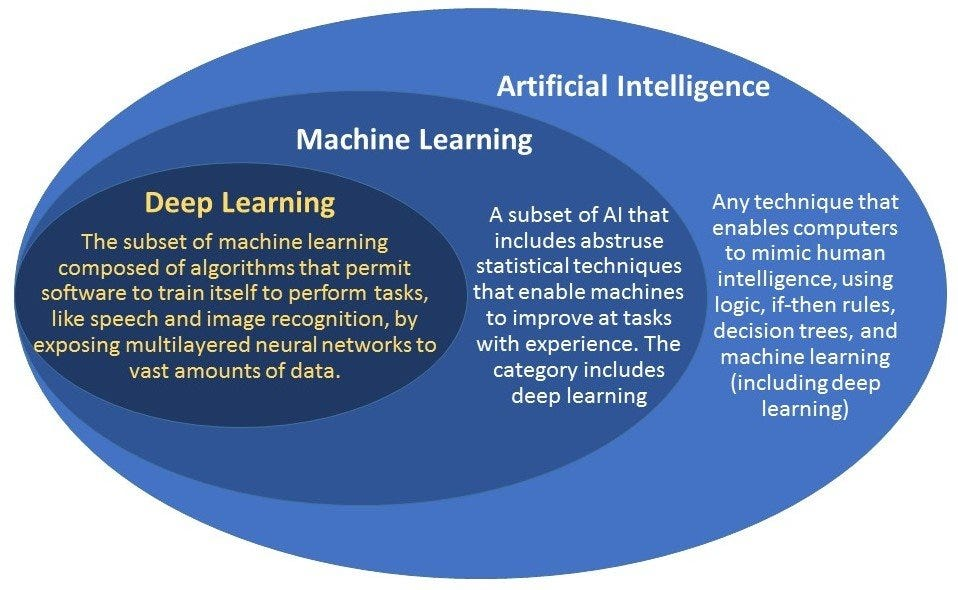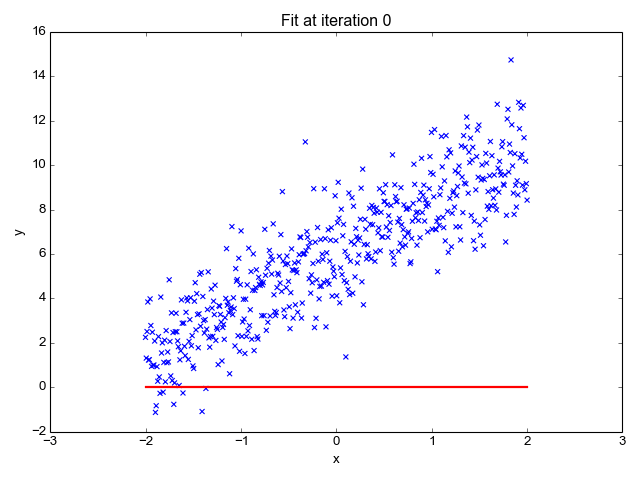medium.com Go back Open original

# Machine Learning for Anyone Who Took Math in Eighth Grade

By Kyle Gallatin

I usually see artificial intelligence explained in one of two ways: through the increasingly sensationalist perspective of the media or through dense scientific literature riddled with superfluous language and field-specific terms.

There’s a less publicized area between these extremes where I think literature needs to step up a bit. News about “breakthroughs” like that stupid robot Sophia hype up A.I. to be something akin to human consciousness while in reality, Sophia is about as sophisticated as AOL Instant Messenger’s SmarterChild.

Scientific literature can be even worse, causing even the most driven researcher’s eyes to glaze over after a few paragraphs of gratuitous pseudo-intellectual trash. In order to accurately assess A.I., the general population needs to know what it really is. And all it takes to understand fundamental A.I. is some middle school math. I may be prone to oversimplification — and I’ll ask all my math, data science, and engineering colleagues to bear with me as I do it — but sometimes that’s what pretentious science needs.

Quintessential, classic A.I. is anything that mimics human intelligence. This could be anything from video game bots to Sophia to sophisticated platforms like Deepmind’s Alphago.Ignore ‘deep learning ’— in this context, it’s the same. Chart: Geospatial World

Machine learning is a subset of A.I. that allows machines to “learn” from real-world data instead of acting on a set of predefined rules.

But what does “learn” mean? It might not be as futuristic as it seems.

My favorite go-to explanation is that machine learning is just y=mx+b on crack. If you’re watching anything like Black Mirror, it’s pretty easy to start to visualize modern A.I. as a conscious entity — something that thinks, feels, and makes complex decisions. This is even more prevalent in the media where A.I. is consistently personified and then likened to Terminator’s Skynet or The Matrix.

We can have a computer look at the input (x) and the output (y) and figure out what ties them together.

In reality, that’s not true at all. In its current state, A.I. is just math. Sometimes it’s difficult math, and sometimes it requires extensive knowledge of computer science, statistics, and other fields. But at the end of the day, a modern A.I. is, at its core, just a mathematical function.

No worries if you’re unfamiliar with math functions because you don’t remember or use them. To grasp this, we only need the easy stuff: There is input (x), and there is output (y), and the function is what happens between the input and output — the relationship between the two.

Super-simplified A.I. is a function expressed as y=mx+b. We already know x and y; we just need to find m and b to understand what the relationship between x and y is. For example, in the table below, x is the input and y is the output.

For this pattern, in order to get y from x we need to multiply x by 1 (giving us the m value) and add 1 (giving us the b value). And so, the function is y=1x+1.

There you go. We found m=1 and b=1. We just took some data (the table above) and created a function that described it. In essence, that is what machine learning is. Using input x, we made a prediction of what y would probably be for all examples.

The fancy part is how you teach a machine to learn what function best describes the data — but when you’re done, what you’re left with is generally some form of y=mx+b. Once we have that function, we can also plot it on a graph:

For more explanation of functions, Math Is Fun has an intuitive and straightforward site (even if the name is a potential red flag and the site looks like their web designer quit sometime in the early 2000s).

Obviously, y=1x+1 is a really simple example. The whole reason we have machine learning is because humans can’t look at millions of data points and come up with a complex function to describe the output. Instead, we can train a computer to look at the input (x) and the output (y) and figure out what ties them together.

In any case, there must be enough data for a correct function to be found. If we only have one data point for x and y, neither we nor a machine could predict only one accurate function. In the original example where x=1 and y=2, the function could be y=2x, y=x+1, y=([x+1]*5–9)⁵ + 1, or any number of possibilities. If we don’t have enough data, the function we have our machine create is liable to have a ton of error when we try and use it on more data.

Also, real-world data isn’t always so perfect. In the example below, a machine has determined several functions that fit most of the data — but sometimes the lines don’t pass through every point. Unlike the old tables from math class, data collected from the real world is more unpredictable, and can never be described perfectly.This is a basic example of a machine learning a function that best represents the data. Chart via Towards Data Science

Finally, the last thing humans can’t do is look at a bunch of variables. It’s easy with just x and y, but what if there isn’t only one input variable? What if y is affected by x¹, x²,…x¹⁰⁰. Very quickly functions can become increasingly complex (for humans).

Let’s look at a real-world example. I work in pharmaceuticals, so let’s say we have a cancer-related dataset that has two input variables on tumor sizes — radius and perimeter — and two potential outputs for whether or not the tumor is benign or metastatic (potentially life-threatening). It may seem complicated, but we need only apply the familiar y=mx+b concept:

• y is the diagnosis and can be 0 (benign) or 1 (metastatic).
• x2 is the perimeter.
• Each x has an unknown m; let’s call them “something.”
• b stays the same as the unknown constant.
Of course, even the most detailed, multi-factored data isn’t perfect, and therefore our machine learning model won’t be either.

How does our linear equation look now? Not much different from the example above:

diagnosis = (something1*radius) + (something2*perimeter) + b

As I explained above, we’re getting out of the realm of human capability. So instead of looking at the data and trying to find out what something we have to multiply our variables by to get an accurate estimate of diagnosis, we have machines do it for us. And that is machine learning!

Of course, even the most detailed, multi-factored data isn’t perfect, and therefore our machine learning model won’t be either. But we don’t need it to be right 100 percent of the time. We simply need it to come up with the best possible function it can that’s right most of the time.

This piece only scratches the surface of the incredible math and computer science that goes into machine learning. But even at complex levels, the concept is the same. No matter how wildly impressive or ridiculous machine learning and A.I. may seem, it all stems from functions a machine has learned to best describe data.